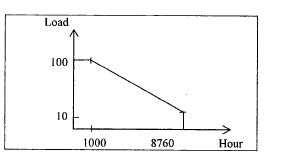Courses

# Test: Power Systems - 7

## 25 Questions MCQ Test Electrical Engineering SSC JE (Technical) | Test: Power Systems - 7

Description
This mock test of Test: Power Systems - 7 for Electrical Engineering (EE) helps you for every Electrical Engineering (EE) entrance exam. This contains 25 Multiple Choice Questions for Electrical Engineering (EE) Test: Power Systems - 7 (mcq) to study with solutions a complete question bank. The solved questions answers in this Test: Power Systems - 7 quiz give you a good mix of easy questions and tough questions. Electrical Engineering (EE) students definitely take this Test: Power Systems - 7 exercise for a better result in the exam. You can find other Test: Power Systems - 7 extra questions, long questions & short questions for Electrical Engineering (EE) on EduRev as well by searching above.
QUESTION: 1

Solution:
QUESTION: 2

Solution:
QUESTION: 3

### Consider the following for line conductors: 1. Hard drawn copper 2. Cadmium copper 3. Aluminum 4. Galvanized steel The correct sequence of the descending order of their electrical conductivities is

Solution:
QUESTION: 4

Lighting arresters are used in power systems to protect electrical equipments against

Solution:
QUESTION: 5

The voltages across the various discs of a string of suspension insulators having identical discs is different due to

Solution:
QUESTION: 6

A Bucholz relay is used for

Solution:
QUESTION: 7

To prevent maloperation of differentially relay while energizing a transformer, the relay restraining coil is biased with

Solution:
QUESTION: 8

In a medium or high head hydroelectric power station, a surge tank is provided to

Solution:
QUESTION: 9

Transmission lines are transported to

Solution:
QUESTION: 10

The impedance value of generator is 0.2 pu on a base value of 11 kV, 50 MVA. The impedance value for a base value of 22 KV, 150 MVA is

Solution:
QUESTION: 11

A surge voltage of 1000 kV is applied to an overhead line with its receiving -end open. If the surge impedance of the line is 500W, then the total surge power in the line is

Solution:
QUESTION: 12

Impulse ratio of insulators and lightning arresters should be

Solution:
QUESTION: 13

Bundled conductors in EHV transmission system provide

Solution:
QUESTION: 14

The charging current in a transmission line increases due to corona effect because corona increases

Solution:
QUESTION: 15

If the fault current is 2 kA, the relay setting is 50% and the C.T. ratio is 400/5, then the plug setting multiplier of a relay will be

Solution:
QUESTION: 16

The load curve of system is shown in the figure.The load factor of the system is

Solution:
QUESTION: 17

Control rods used in neuclear reactors are made of

Solution:
QUESTION: 18

In a 3-core extra-high voltage cable, a metallic screen around each core-insulation is provided to

Solution:
QUESTION: 19

Galloping in transmission line conductors arises generally due to

Solution:
QUESTION: 20

Hollow conductors are used in transmission line to

Solution:
QUESTION: 21

Zero sequence current can flow from a line into a transformer bank if the windings are in

Solution:
QUESTION: 22

When a line-to-ground fault occurs, the current in a faulted phase is 100 A. The zero sequence current in this case will be

Solution:
QUESTION: 23

"Expanded ACSR" are conductors composed of

Solution:
QUESTION: 24

The non-uniform distribution of voltage across the units in a string of suspension type insulators is due to

Solution:
QUESTION: 25

Whenever the conductors are dead-ended or there is a change in the direction of transmission line, the insulators used are of the

Solution: